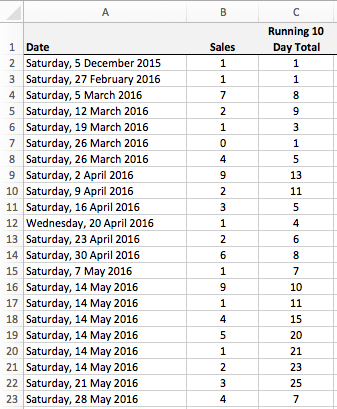# Use SUMIFS to calculate a running total between two dates

This lesson shows you how to calculate a running total for a table of data that based on the dates in the table. An example might be calculating a running total of sales for the last 30 days, although you could use any date range you like, including future dates.

We've already covered how to create a running total in Excel (all links in this lesson open in a new tab)\$, but that lesson assumed that the running total always has the same starting point, i.e. the first value in the table.This time, we want to calculate a running total for a given date period. e.g. total sales for the last 10 days. With this calculation, the sales figure will vary each day, depending on the sales made in the previous 10 day period.

Here's an example of a spreadsheet that we'll use to build a formula to do what we need. As you can see, we already have a running total that includes the previous 10 days of sales data for each row. For example, the running total for April 30 is 8, which includes all sales for the period from April 21 up to and including to April 30.In the next section, we will look at how these numbers were calculated.

## Use the SUMIFS function to create a running total based on a date range

The SUMIFS function allows us to add up numbers in a table based on multiple criteria. As such, it is perfect for our requirements in this scenario.

Remember that the syntax for the SUMIFS function is as follows:

=SUMIFS(sum_range, criteria_range1, criteria1, criteria_range2, criteria2,....)

Here's how we'll construct the SUMIFS function:

• The Sum_range is B2:B23.
• Both Criteria_range1 and Criteria_range2 are A2:A23.
• Criteria1 checks if the value in the criteria range is less than, or equal to, the date in the current row. In other words, if we're calculating the running total up to 7 May, we only want our running total to include numbers up to and including 7 May.
• Criteria2 checks if the value in the criteria range is greater than the current date minus the number of days we are including in our running total. In other words, we would only want to include the 10 days up to and including 7 May.

Fron that, we can construct the formula for C2. Once we have it working correctly in C2, it can be copied and pasted to the other cells in column C.

=SUMIFS(\$B\$2:\$B2,\$A\$2:\$A2,"<="&A2,\$A\$2:\$A2,">"&A2-10)

Let's look more closely at the components of this function. Notice the use of absolute and mixed references - this is an important part of the formula.

• The sum_range is \$B2\$2:\$B2. When we paste this formula down the table, the range will change, i.e. \$B2\$2:\$B3, \$B2\$2:\$B4, etc.
• Similarly, the criteria_range is \$A\$2:\$A2. This is so our formula behaves correctly when we get to rows 16-21, each of which contain a sales figure for May 14. If we specified a criteria_range of \$A\$2:\$A\$2, the total for rows 16-17 would be the same, rather than increasing in each row as they do.
• Criteria_1 and Criteria_2 both use concatenation to construct the criteria. This allows us to construct dynamic criteria
• Criteria_1 makes sure we only sum values from rows where the date is less than or equal to the date in column A for that row. For example, criteria_1 in row 10 allows only dates up to and including April 9
• Criteria_2 makes sure we only sum values from rows where the date is greater than 10 days before the date in column A. So criteria_2 in row 10 allows only dates after March 30.
• Here's the crucial part - these two criteria work together so that only dates that meet both criteria (after March 30, and up to April 9) are included in the calculation.

## Summary

The SUMIFS function provides a powerful and relatively easy way to calculate a running total in a table of data where you want the total to reflect a dynamic date range, such as "all sales in the last 10 days" or "all expected expenditure in the next 30 days".

## Our Comment Policy.

Add a comment to this lesson

### Assistance with formal setup

How do I add a column that has a certain code in another column for all entries within a given month?

For example from my yearly tally I want to find out the total spent on food for each month separately.

I am using Excel on a Mac

### How can I add a ROW counter?

Hi.

I use SUMIFS to calculate a cumulative total of time spent on different work projects. The formula is:

=SUMIFS([Session Hours],[Date],"<="&\$A2,[Project],"="&\$C2)

Where column A is the Date field, and column C is the Project field. (Sample attached.)

But I have a problem. I frequently work on the same project in two different sessions on the same day - and my SUMIFS function adds all sessions to the *end* of that day, not just to the current row.

I tried various ways of adding in a condition based on the ROW function, but Excel always claimed there was an error in the formula (there wasn't as far as I could tell). Then I tried adding in an INDEX function to specify that the range to be summed went from row 1 of the table to [@Current Row]. This returned a "VALUE" error message.

So, I'm stumped... And ideas?

Many thanks,

Richard

### Multiple selection to sum value over a date range

I would like to select a Purchase order number to return from a second sheet all cost invoiced with in a date range associated with the purchase order number. The data source has a column containing all purchase order numbers, cost invoiced for the purchase order by the date. Therefore there can be multiple row with same purchase order invoiced on different dates with different amounts. Therefore the result on a summary sheet would show the total amount invoiced for example may 1st 2017 to May 31st 2017 for each unique purchase order.